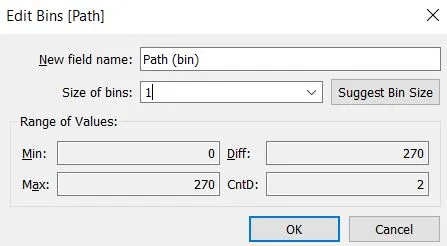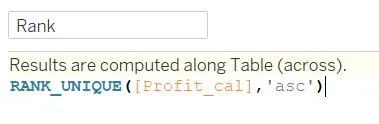top of pageSearch

# A step-by-step guide on how to plot a Radial Chart in TableauA radial chart is another variation of a bar chart displayed on the polar coordinate system instead of the cartesian system. Like any other chart in tableau, the Radial chart is another fascinating Chart and easy to interpret details for the user. The radial diagram visualizes the relationship of each item that is linked to the central item. It uses different arcs to compare different categories.

Without further delay let us get into the chart.

We will start by loading the Sample superstore data into Tableau. Here we are going to visualize Subcategory-wise Profit using Radial Chart.

Step 1:Step 2:

Calculated Fields

We have to create the following calculated fields to create the radial chart

Path: To create a Radial chart we need two points, the start point, and the endpoint. Here 0 and 270 are the start and the endpoints respectively.Path bin: This helps to create evenly spread data points between the start and end points (i.e 0 and 270). Create a path bin and edit the size of bins as 1.Index: -1 is given because the first row of the index starts from 1 but the path value is from 0.Profit_cal: To find the profitRank: Helps to create a different arc for different subcategories.Total Profit: To find the profit for each subcategory.Percentage: To Calculate the Percentage of the Profit.Size: Helps to change the size of the arc based on the value.X:We need X and Y points to draw the linesY:Step 3:

Plotting the Graph

Drag and drop X to Column and both Y and Path Bins to Row. In path-bins make sure the ‘show missing value’ is selected.

Change the marks to line and move the path bins from Row to line in the marks and put Sub-category in colors.Edit Table Calculation to get the proper shape of the Radial Chart

For X

• Right-click on X in the Rows and select compute using Path(bin)

• Again Right click on X and select Edit Table Calculation

In Nested Calculations

For index: Select only path(bin)

For Size: Select both Path(bin) and Sub-category then drag and put Path(bin) below Sub-category.

For Total Profit: Select both Path(bin) and Sub-category then drag and put Path(bin) below Sub-category.

For Rank: Select only Sub-Category.

For Y

• Right-click on Y in the Columns and select compute using Path(bin)

• Again Right click on Y and select Edit Table Calculation

In Nested Calculations

For index: Select only path(bin)

For Size: Select both Path(bin) and Sub-category then drag and put Path(bin) below Sub-category.

For Total Profit: Select both Path(bin) and Sub-category then drag and put Path(bin) below Sub-category.

For Rank: Select only Sub-Category.

Now the chart will look like thisStep 4:

Formatting the Chart for better Visualization

• Drag and drop Sub-category and Percentage to Label.• Right-click on Label in marks and edit Label Appearance

• Again Right click on Label, in ‘Marks to label’ choose Line Ends, and in Options deselect ‘Label End of Line’.• Format the Percentage in marks to get the display of percentage value in the chart.

• Increase the Size in marks for the thicker appearance of the arcs.

Here is the final outcome of our Radial chartHere is a basic Radial chart I have created using Tableau. I tried to make it as simple as possible. Hope this blog will be useful for creating a Radial chart with these easy steps. Thanks for Reading!!!